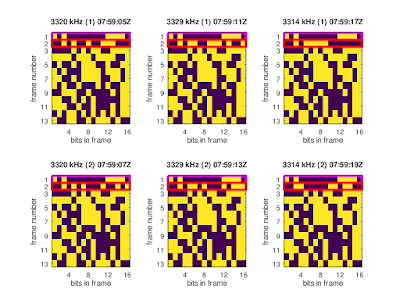## Sunday, September 8, 2019

### 8-ary constellation bursts at 12800 bps data rate (2)

This is a follow-up to this blog post.

With the help of kiwirecorder.py a number of bursts were recorded (the -T option helps to save disk space) and then decoded using gr-digitalhf.

## 3-channel mode

At the time of observation, the signals were active on fA=3320 kHz, fB=3314 kHz, and fC=3329 kHz only, and the schedule was [(A,B,C), (A,B,C), ...]. On each frequency two consecutive bursts were observed, with the preambles exactly 2 seconds apart.

### Shortened preamble for the 1st burst

The preamble for the 1st burst on each frequency is shortened w.r.t. nominal STANAG 4539 preamble:S4539 preambles for the 1st and 2nd burst

### The payload data is mostly the same within each (A,B,C) cycle

Arranging the 104 WALSH-encoded payload di-bits in frames of size 13×16, the plots below were obtained and the following observations can be made:
• Except for the 2nd frame (marked in red) all other bits are the same within each (A,B,C) cycle
• The parity of the 1st frame (marked in magenta) and of the 2nd frame is even
• The parity of the 11×16 bits in frames 3-13 is even
• Analyzing the bit stream obtained by concatenating 1st frames (omitting repetitions) it can be found that the rank deficiency for k=16 is 5, i.e, besides the one parity bit there are four more bits being used for error correction
• Similarly the rank deficiency for concatenated 2nd frames at k=16 is 7. As the 1st two bits of each 2nd frame seem to be zero this might indicate a similar error correction for the 1st and the 2nd frame.1st (A,B,C) cycle2nd (A,B,C) cycle

### Content of the 2nd frame

The 1st 8 bits of the 2nd frame seem to contain a (6-bit wide) counter:
• the period of this counter is 30, i.e., after 5 (A,B,C) cycles, corresponding to 100 seconds, the sequence of count values repeats
• the start times for the bursts mod 100 seconds repeat after 30 bursts as well
• this counter is related to mod(number of the current UTC second, 100), see the plots below
• the following is pure speculation: the current difference between TAI (International Atomic Time) and UTC (Coordinated Universal Time) is 37 seconds, so this counter×2 may be related to mod(TAI seconds, 100)1st 8 bits of the second frame; the black areas indicate missing data

## 6-channel mode

The same observations are true when the system is using 6 HF channels, e.g.,
fA1=4024 kHz, fB1=4030 kHz, and fC1=4039 kHz and
fA2=4784 kHz, fB2=4790 kHz, and fC2=4799 kHz
The sequence of transmissions in this case was
[(A2+C2), (A1+B1), (A2+B2), (B1+C1), (B2+C2), (A1+C1)], ...
i.e., two signals were active at the same time. The channels A1,B1,C1 were broadcasting two bursts each and A2,B2,C2 one burst. Also here the same payload data is used for all 12+6 bursts in one cycle, and the 2nd frame in each packet carries a number related to the time of transmission as shown above.